# Introduction to Graphing

Introduction to Graphing

In this section, we will understand the graphical significance of a two-variable linear equation. Consider the equation $$x + 2y = 4$$. Let us write down a few specific solutions of this equation:

 $$x=$$ $$0$$ $$1$$ $$2$$ $$3$$ $$4$$ $$y=$$ $$2$$ $$\frac{3}{2}$$ $$1$$ $$\frac{1}{2}$$ $$0$$ $$sol$$ $$\left( {0,2}\right)$$ $$\left({1,\frac{3}{2}} \right)$$ $$\left( {2,1}\right)$$ $$\left({3,\frac{1}{2}} \right)$$ $$\left( {4,0}\right)$$

Let us plot these five solutions (points) on a coordinate plane: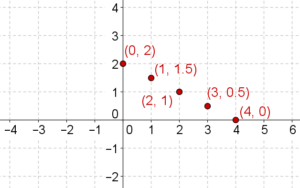These five points seem to be collinear, that is, they seem to lie on a straight line. In fact, they are collinear! And not just these five points: if you take any other solution of this equation, it will also lie on the same straight line: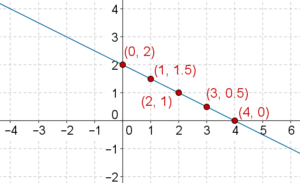And, every point on this particular straight line will satisfy this particular linear equation. Note: when we say that a point satisfies a linear equation or a point is a solution of a linear equation, what we mean to say is that the coordinates of the point satisfy the linear equation.

Let us summarize: any two-variable linear equation (say E) graphically corresponds to a straight line (say L) in the coordinate plane. Every solution of E lies on L, and every point on L is a solution of E. Any point not on L is not a solution of E.

In a higher class, you will clearly understand why the graph of a linear equation is a straight line. For now, just observe the fact that the word linear comes from the word line. Since any equation of the $$ax + by + c = 0$$ graphically corresponds to a straight line, that is why it is called a linear equation.

To uniquely plot a line, we need only two points lying on it. Thus, to plot the graph of a linear equation, we need to plot only two distinct solutions of the equation. And then, because we know that the graph of a linear equation is a straight line, we can simply draw the line through these two points to obtain the graph of the equation.

Let us apply this to a few examples.

Example 1: Plot the graph of the linear equation $$x - 4y + 3 = 0$$.

Solution: We determine any two solutions of this equation:

 $$x =$$ $$1$$ $$- 1$$ $$y =$$ $$1$$ $$\frac{1}{2}$$ $$sol$$ $$\left( {1,1}\right)$$ $$\left( { -1,\frac{1}{2}} \right)$$

We plot these two solutions, and then draw the line through them to obtain the graph of the given equation: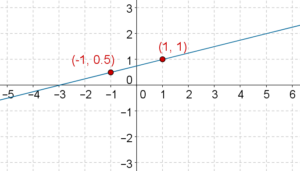Let us assure ourselves that this is indeed the graph of $$x - 4y + 3 = 0$$. For that purpose, we have picked three points on this line other than the two solutions we used to plot the line: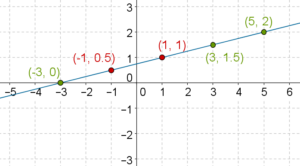You may verify that these three points are also solutions of $$x - 4y + 3 = 0$$. In fact, if you take any other point whatsoever on this line, it will be a solution of $$x - 4y + 3 = 0$$. And, any point not on this line will not be a solution of $$x - 4y + 3 = 0$$. You should proceed further only when you are absolutely clear about this discussion.

Example 2: Plot the graph of $$- \frac{1}{2}x + \frac{1}{3}y = 1$$.

Solution: We determine any two specific solutions of the equation:

 $x =$ $$2$$ $$0$$ $y =$ $$6$$ $$3$$ Sol $\left( {2,6}\right)$ $\left( {0,3}\right)$

The graph of the equation is plotted below: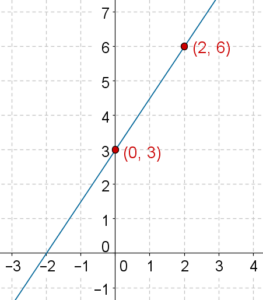Example 3: The graph of a linear equation is presented below: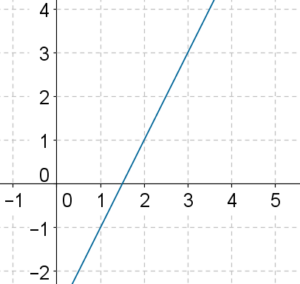Which of the following equations does it represent?

(A) $$x - 2y + 4 = 0$$

(B) $$2x + y - 3 = 0$$

(C) $$x + 2y + 1 = 0$$

(D) $$2x - y - 3 = 0$$

Solution: We take any two points on the graph, and determine that equation which both these points satisfy: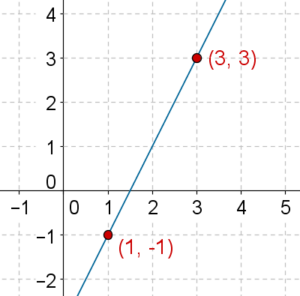Now, it is easy to verify that among the given options, the only equation which both these points P and Q satisfy is $$2x - y - 3 = 0$$. Thus, the correct option is (D).

Example 4: In the figure below, three equations are specified, and three lines are drawn. By inspection, determine which equation corresponds to which line.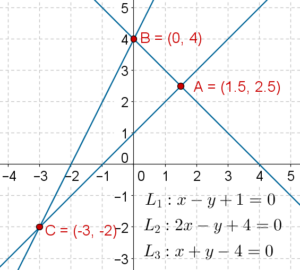Solution: By inspection, you may note that

-  $$A,\,B$$ satisfy $${L_3}$$

-  $$B,\,C$$ satisfy $${L_2}$$

-   $$C,\,A$$ satisfy $${L_1}$$